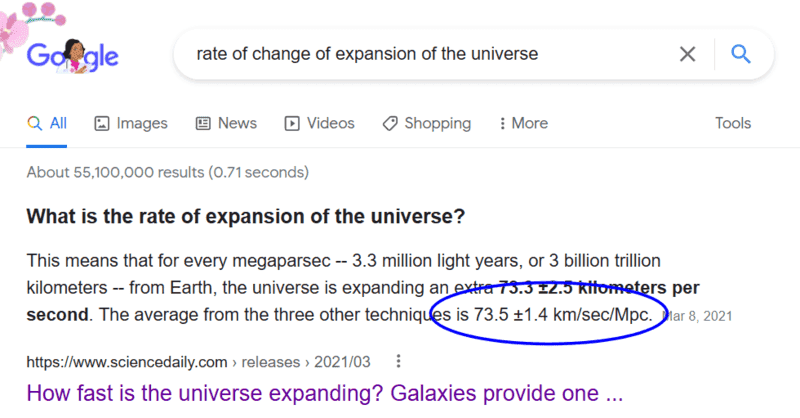# What is the acceleration of the expansion of the Universe?

• I
• JamesGarderiner

#### JamesGarderiner

TL;DR Summary
I am aware that the universe expansion is accelerating and I have heard that the acceleration might not be constant. I need values of the acceleration and rate of change of acceleration and I can't find any.
I can't find any values of acceleration or rate of change of acceleration of the expansion of the universe when I looked it up and I need these values for a theory I'm working on that could supersede dark energy and show the universe is closed even if everything accelorating away from us and even if the rate of change of acceleration is positive. Does anybody know these values or know where I might be able to find them? Thanks :)

Summary:: I am aware that the universe expansion is accelerating and I have heard that the acceleration might not be constant. I need values of the acceleration and rate of change of acceleration and I can't find any.

Does anybody know these values or know where I might be able to find them?
https://en.wikipedia.org/wiki/Expansion_of_the_universe

Summary:: I am aware that the universe expansion is accelerating and I have heard that the acceleration might not be constant. I need values of the acceleration and rate of change of acceleration and I can't find any.

I can't find any values of acceleration or rate of change of acceleration of the expansion of the universe when I looked it up and I need these values for a theory I'm working on that could supersede dark energy and show the universe is closed even if everything accelorating away from us and even if the rate of change of acceleration is positive. Does anybody know these values or know where I might be able to find them? Thanks :)
What it means for the universe expansion to be accelerating is explained here:

https://blackwells.co.uk/bookshop/p...ology-by-Andrew-R-Liddle-author/9781118502143

A bargain at £24.99.

I can't find any values of acceleration or rate of change of acceleration of the expansion of the universe
I can only conclude that either you didn't look very hard at all or you somehow didn't understand the answer when it was presented. This is what I got with a simple search•Motore and berkeman
I can only conclude that either you didn't look very hard at all or you somehow didn't understand the answer when it was presented. This is what I got with a simple search
73.5 km/sec/Mpc shows how the velocity of bodies relative to us changes with distance, If a star was 1 Mpc away from us it would be going 73.5 km/s. That's velocity, not acceleration nor rate of change of acceleration. How dare you slander me sir! If you were to read the question that the answer is for, it says the rate of expansion, not the rate of change of expansion, which is what you searched. That's why I'm having difficulty. Everywhere says the rate of expansion but non say the rate of change of expansion!

•weirdoguy
What it means for the universe expansion to be accelerating is explained here
I know what it means for the universe expansion to be accelerating, however, I can't find a magnitude of this acceleration. That's what I meant by the question.

73.5 km/sec/Mpc shows how the velocity of bodies relative to us changes with distance, If a star was 1 Mpc away from us it would be going 73.5 km/s. That's velocity, not acceleration nor rate of change of acceleration. How dare you slander me sir! If you were to read the question that answer is for, it says the rate of expansion, not the rate of change of expansion, which is what you searched. That's why I'm having difficulty. Everywhere says the rate of expansion but non say the rate of change of expansion!
I didn't slander you, I offered two possibilities. You have shown that my second one was correct. You didn't understand the answer. You are looking for something that doesn't exist**. If it DID exist, the statement in Wikipedia would be incorrect and presumably would not be there (or in the hundreds, perhaps thousands, of other places you can find it, including textbooks.

@berkeman gave you a link in post #2 which explains the expansion.

**I'm assuming you mean rate of change of acceleration over time, in the sense that something a given distance D away from us is accelerating away from us now at rate X but that at some time in the future an object a distance D away from us will be accelerating away from us at a rate of nX and you are looking for n.

••Bandersnatch and berkeman
I need these values for a theory I'm working on
Discussion of personal theories is off topic for Physics Forums. Thread closed.